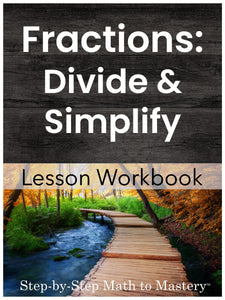# Fractions | Dividing Fractions and Simplifying Fractions

• \$12.00
Unit price per
Shipping calculated at checkout.

## Dividing Fractions and Mixed Numbers (with Word Problems) | Simplifying or Reducing Fractions

### 22 Step-by-Step Math Lessons | Special Education Math Intervention | Fifth Grade Math Level

This Dividing Fractions and Simplifying Fractions lesson workbook includes an example IEP goal, 22 step-by-step math lessons, reviews and assessments. This evidence-based math intervention is tied to fifth grade standards and is great for special education math goals and tier 2 small group math interventions (RTI).

These sequential lessons are easy to teach, with enough material for 5 weeks of instruction!

Divide Fractions

In this workbook, students will learn to rewrite fraction division problems as multiplication problems.  The “invert and multiply” strategy is a reliable one that can be used with fractions and mixed numbers.

Simplify Fractions

These lessons cover two main topics:  divisibility rules and simplifying fractions by cancelling prime factors.

22 Lessons
4 Reviews
4 Tests

146 pages

5 Weeks of Instruction

## Students Will Be Able To:

Divide Fractions

• Read and write fractions as division problems
• Write division problems as fractions
• Write reciprocals
• Divide whole numbers by fractions
• Divide fractions by whole numbers
• Divide fractions by fractions
• Solve word problems involving dividing fractions
• Divide mixed numbers by whole numbers
• Divide mixed numbers by mixed numbers

Simplify Fractions

• Use divisibility rules to determine whether a number can be divided evenly by 2, 3, or 5.
• Identify simpler fractions
• Multiply more than two factors
• Identify prime numbers
• Find the prime factors of a number using a factor tree
• Rewrite fractions using prime factors
• Simplify fractions by cancelling common factors

### Lessons Are Tied to These Fifth and Sixth Grade Math Standards:

• Interpret a fraction as division of the numerator by the denominator (a/b = a ÷ b). Solve word problems involving division of whole numbers leading to answers in the form of fractions or mixed numbers (CCSS 5.NF.B.3)
• Divide unit fractions by whole numbers and whole numbers by unit fractions. (CCSS 5.NF.B.7)
• Interpret and compute quotients of fractions, and solve word problems involving division of fractions by fractions (CCSS 6.NS.A.1)

### Teacher Feedback:

❤️ “These materials are a MUST for special education teachers. Fractions are difficult for a lot of students and this pacing and practice helps them to finally "get it" and be able to move on with confidence in their learning." -Tracey M.

❤️ “Love! Provided repetition and visuals that students with learning disabilities need." -Debra Z.

❤️ “This resource is great for Special Education students. I've used it for planning lessons for this next year and I am excited to teach them. The material is so much easier to understand than what I have used previously." -Kari E.

❤️ “It really breaks the concept down and helps students understand fractions." -Tonya T.

❤️ My students love this program. They keep begging to do more pages in the packet :) It makes them feel successful in math class and boosts their confidence! Thank you!!" -Lisa L.

❤️ “I used this to teach my tier 3 math intervention students in grade 7. I loved the option of no cute clip art so it didn’t look babyish for my students. The progression from concrete to pictorial to abstract was perfect. My students felt more confident with fractions." -Sue W.

❤️ “I firmly believe there is no better math resource out there. This workbook is MIRACULOUS. No other videos, tutorials, or books come close to what this workbook offers - peaceful, easy learning. Amazing!" -Jennifer D.

❤️ “Amazing!!! So well thought out. There are so many opportunities for the students to practice their new skills too." -Jennifer L.

### Not sure where to start?

Watch a video overview of Step-by-Step Math to Mastery or read the transcript here.

### Step-by-Step Math to Mastery™ Resources:

Make math easier to understand.

• Help students over math hurdles with clear, sequential, scaffolded lessons.
• Prevent overwhelm. Build student confidence.

Make math easier to teach.

• Save hours of planning and piecing together materials.
• Paraeducators can deliver quality instruction independently. Open & teach.

### Reasons You'll Love Step-by-Step Math to Mastery™ Resources

• Boosts student confidence and progress
• Time-saving: print and teach
• Easy prep: only black ink is needed
• Easy to teach, paraeducator-friendly
• Example IEP goals and shorter term objectives
• Consistent & predictable format
• Lots of practice repetitions
• Scaffolded with structured workspaces
• Fewer problems on a page, white space, minimal visual clutter
• Tied to standards
• Explicit/Direct instruction
• Systematic & sequential
• Mastery approach--teaching one topic at a time, one strategy at a time
• Lessons have "I Can" statements, model problems, guided practice, & independent practice
• Each workbook can be used individually as a stand-alone intervention for that skill or they can be used together, taking students from the basics of number sense and addition in first grade all the way to dividing fractions and decimals in fifth grade.
• Can also be used with older students (middle & high school) to help fill the gaps in their learning.